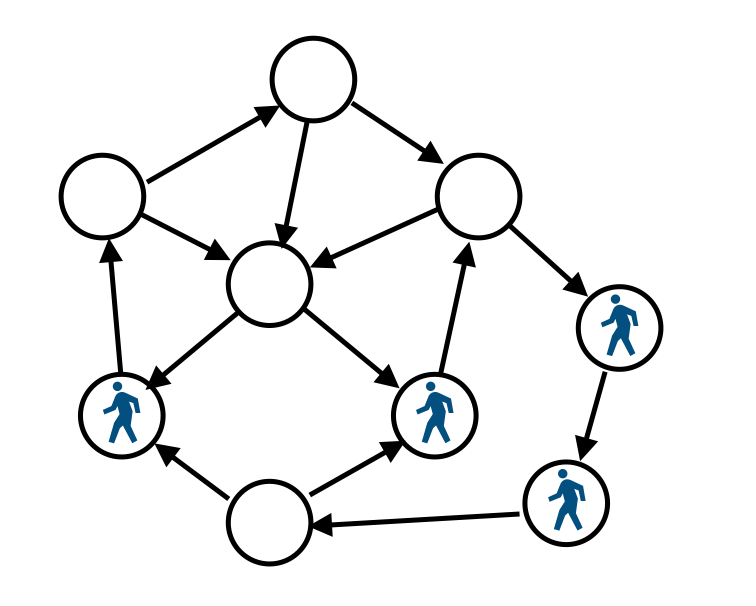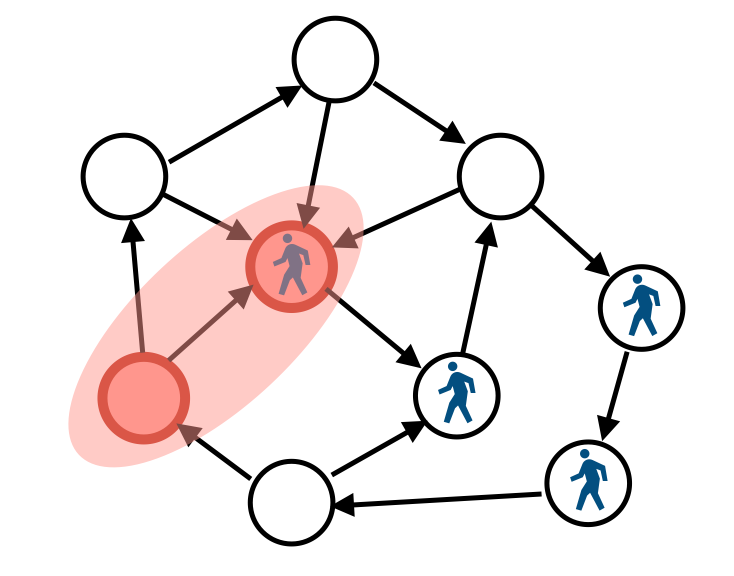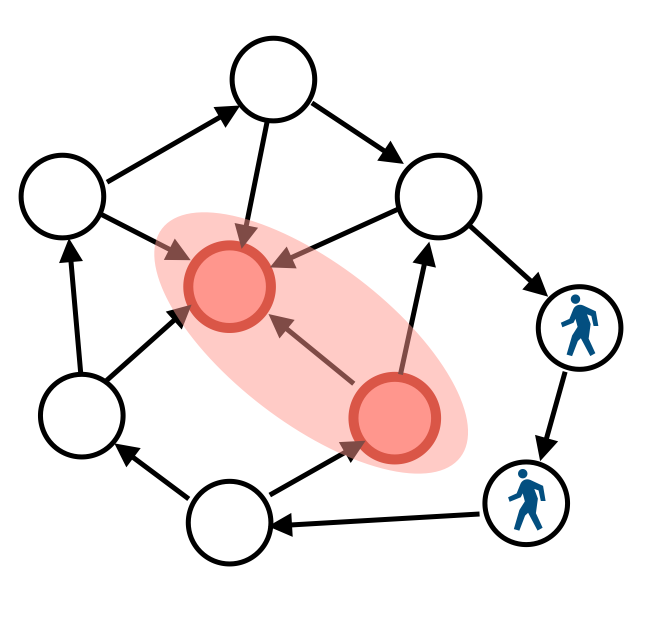# AtCoder Grand Contest 035. B. Even Degrees

We can restated the problem as follows. There are people on vertices with odd degree. When we swap the direction of the edge, the human on the end-points moves on the edge. If both endpoints have people, these two will disappear. We want to remove all the people.It is easy to remove them. We just move all the people to one place. If there are the odd number of people, we cannot construct because one human remains.

# Codeforces Round #573 (Div. 1) D. Tokitsukaze and Strange Rectangle

https://codeforces.com/contest/1190/problem/D

If there is a point on the boundary of the rectangle, strictly speaking, this point is not contained in the rectangle, but we can consider it is contained in. The reason why we can include it by slightly expand the rectangle. Each the left side, right side and bottom side of the rectangle has at least one point on itself. The reason why we can shrink the rectangle until collides some points.

In this condition, there exists at least one point on the bottom side, then focus on the left-most point among them.

Let us count the rectangles such that point P is on its bottom side and is the left-most point. If we can solve this problem, we can obtain the answer by summing up the answer for each P.

To solve this problem, we should know the number of possible left sides and right sides, respectively. Line sweep and BIT will be useful.

# Codeforces #573 (Div. 1) C. Tokitsukaze and Duel

https://codeforces.com/contest/1190/problem/C

We can return the same state by doing the same action. If we will be defeated, we should give them the same state. Therefore, this game will not end.

However, we cannot imitate the first action. If the first player can immediately defeat the other, the first player will win. If the second player can win after for any action, the second player will win. Otherwise, this game will end draw.

# AtCoder Beginner Contest 133 F Colorful Tree

There are several solutions. If we may use wavelet matrix, this task becomes very easy. This method works in the online version, that is, we can reply the answer efficiently if we can't know the next query before do the previous one.

Wavelet matrix supports the 2-dimensional summation query. Here, I explain what a wavelet matrix can do. A wavelet matrix consists of n points (x[i], y[i]). Each point has some integer value. We specify the rectangle [l, r) * [b, t), then the wavelet matrix returns the sum of values in this rectangle.

In this problem, we define the i-th point as (hld.label[i], color[i]) and the value as the weight between i and its parent. With heavy light decomposition, we can figure out the total distance of u-v only using specified color. Hence, we can solve this problem O(n + q log^2 n), which is fast enough.

screencast

In the following code, wavelet matrix is simplified for this problem. More generic version is here Submission #6309154 - AtCoder Beginner Contest 133, but it is slower than simplified one. Perhaps, we can optimize it more, I didn't try any optimization. By the way, this wavelet matrix is not verified enough. I can't remember when I used it last, maybe I have never used it.

#include <bits/stdc++.h>

#define rep(i, n) for (int i = 0; i < (n); i++)
#define repr(i, n) for (int i = (n) - 1; i >= 0; i--)

using namespace std;
using ll = long long;

struct HLD {

HLD(const vector<vector<int>> &g) : label(g.size()), parent(g.size()), head(g.size()) {
const int n = g.size();
vector<int> size(n, 1);
auto dfs = [&](auto dfs, int u, int p) -> void {
for (int v : g[u]) if (v != p) {
dfs(dfs, v, u);
size[u] += size[v];
}
};
dfs(dfs, 0, -1);
int k = 0;
auto dfs2 = [&](auto dfs, int u, int p, int h) -> void {
label[u] = k++;
parent[u] = p;
for (int v : g[u]) if (v != p && size[v] * 2 >  size[u]) dfs(dfs, v, u, h);
for (int v : g[u]) if (v != p && size[v] * 2 <= size[u]) dfs(dfs, v, u, v);
};
dfs2(dfs2, 0, -1, 0);
}

int lca(int u, int v) {
for (;;) {
if (label[u] > label[v]) swap(u, v);
}
}

template<class F> void each(int u, int v, F f) {
for (;;) {
if (label[u] > label[v]) swap(u, v);
f(label[u], label[v]);
return;
}
}
}

template<class F> void each_edge(int u, int v, F f) {
for (;;) {
if (label[u] > label[v]) swap(u, v);
if (u != v) f(label[u] + 1, label[v]);
return;
}
}
}

int operator[](int u) {
return label[u];
};
};

// T: ring
template<class T, int H>
struct wavelet_matrix {
vector<vector<int>> cnt;
vector<vector<T>> sum;

wavelet_matrix(vector<pair<int, T>> a) : cnt(H, vector<int>(a.size() + 1)), sum(H + 1, vector<T>(a.size() + 1)) {
auto dfs = [&](auto dfs, int l, int r, int h) -> void {
if (r - l == 0) return;
for (int i = l; i < r; i++) {
sum[h][i + 1] = sum[h][i] + a[i].second;
}
if (h == H) return;
for (int i = l; i < r; i++) {
cnt[h][i + 1] = cnt[h][i] + (~a[i].first >> (H - 1 - h) & 1);
}
int m = stable_partition(a.begin() + l, a.begin() + r, [&](pair<int, T> x) {
return ~x.first >> (H - 1 - h) & 1;
}) - a.begin();
dfs(dfs, l, m, h + 1);
dfs(dfs, m, r, h + 1);
};
dfs(dfs, 0, a.size(), 0);
}

// [l,r) * [b,t)
T query(int l, int r, int b, int t) {
auto dfs = [&](auto dfs, int l, int r, int b, int t, int ll, int rr, int bb, int tt, int h) -> T {
if (r - l == 0 || t - b == 0) return T();
if (tt <= b || t <= bb) return T();
if (b <= bb && tt <= t) return sum[h][r] - sum[h][l];
int mm = ll + cnt[h][rr] - cnt[h][ll];
T vl = dfs(dfs, ll + cnt[h][l] - cnt[h][ll], ll + cnt[h][r] - cnt[h][ll], b, t, ll, mm, bb, (bb + tt) / 2, h + 1);
T vr = dfs(dfs, l + cnt[h][rr] - cnt[h][l], r + cnt[h][rr] - cnt[h][r], b, t, mm, rr, (bb + tt) / 2, tt, h + 1);
return vl + vr;
};
return dfs(dfs, l, r, b, t, 0, cnt.size() - 1, 0, 1<<H, 0);
}
};

int main() {
cin.tie(nullptr);
ios::sync_with_stdio(false);
int n, q;
cin >> n >> q;
vector<vector<int>> g(n);
struct edge {
int v, c, w;
};
vector<vector<edge>> g2(n);
rep(i, n-1) {
int u, v, c, w;
cin >> u >> v >> c >> w;
u--; v--;
g[u].push_back(v);
g[v].push_back(u);
g2[u].push_back((edge){v, c, w});
g2[v].push_back((edge){u, c, w});
}
HLD hld(g);
vector<int> ws(n);
vector<int> cs(n);
auto dfs = [&](auto dfs, int u, int p) -> void {
for (edge e : g2[u]) {
if (e.v == p) continue;
ws[e.v] = e.w;
cs[e.v] = e.c;
dfs(dfs, e.v, u);
}
};
dfs(dfs, 0, -1);
vector<pair<int, int>> val1(n);
vector<pair<int, ll>> val2(n);
rep(i, n) {
val1[hld[i]] = {cs[i], 1};
val2[hld[i]] = {cs[i], ws[i]};
}
wavelet_matrix<int, 17> wm1(val1);
wavelet_matrix<ll, 17> wm2(val2);
while (q--) {
int x, y, u, v;
cin >> x >> y >> u >> v;
u--;
v--;
ll ans = 0;
hld.each_edge(u, v, [&](int l, int r) {
r++;
ans += wm2.query(l, r, 0, 1<<17);
ans -= wm2.query(l, r, x, x+1);
ans += wm1.query(l, r, x, x+1) * y;
});
cout << ans << '\n';
}
}


My first answer is based on: the group of queries having the same color can be performed at once. (My first submission pass the all tests but it is actually wrong. RMQ only takes powers of two but I gave other than them. The code below is already corrected.)
Submission #6303264 - AtCoder Beginner Contest 133

Decompose each query into (root to u), (root to v), (root to lca u v), then traverse tree as computing queries appropriately.
Submission #6310159 - AtCoder Beginner Contest 133

# Codeforces Round #572 (Div. 1) B. Count Pairs

https://codeforces.com/contest/1188/problem/B

\begin{align}
& (x+y)(x^2+y^2)=k \\
\iff & x^3 + x^2 y + x y^2 + y^3 = k \\
\iff & \frac{x^4-y^4}{x-y}=k \\
\iff & x^4 - kx=y^4 - ky
\end{align}

My first approach is to solving cubic equation. I tried to apply Cardano's algorithm for this problem. However, square roots and cubic roots appears in Cardano's method. It is difficult to handle them, so I gave up and tried to find another approach. As a result, I solved this problem but a lot of time is consumed by overthinking.

By the way, can we calculate the cubic roots without discrete logarithm in O(log MOD)? Maybe, this paper states the generic solution for the cubic equations and quadratic equations but I haven't understood anything yet. Cubic and quartic congruences modulo a prime - ScienceDirect

#include <bits/stdc++.h>

#define rep(i, n) for (int i = 0; i < (n); i++)
#define repr(i, n) for (int i = (n) - 1; i >= 0; i--)

using namespace std;
using ll = long long;

int MOD;

struct mint { int n; mint(int n_ = 0) : n(n_) {} };
mint operator-(mint a) { return -a.n + MOD * (a.n != 0); }
mint operator+(mint a, mint b) { int x = a.n + b.n; return x - (x >= MOD) * MOD; }
mint operator-(mint a, mint b) { int x = a.n - b.n; return x + (x < 0) * MOD; }
mint operator*(mint a, mint b) { return (long long)a.n * b.n % MOD; }
mint &operator+=(mint &a, mint b) { return a = a + b; }
mint &operator-=(mint &a, mint b) { return a = a - b; }
mint &operator*=(mint &a, mint b) { return a = a * b; }
istream &operator>>(istream &i, mint &a) { return i >> a.n; }
ostream &operator<<(ostream &o, mint a) { return o << a.n; }
bool operator<(mint a, mint b) { return a.n < b.n; }

int main() {
cin.tie(nullptr);
ios::sync_with_stdio(false);
int n;
mint k;
cin >> n >> MOD >> k;
map<mint, ll> mp;
ll ans = 0;
rep(i, n) {
mint x;
cin >> x;
ans += mp[x*x*x*x - k*x]++;
}
cout << ans << endl;
}